SYLLABUS  Previous: 5.1 Monte Carlo integration  Up: 5 MONTE-CARLO METHOD  Next: 5.3 Particle orbits

## 5.2 Stochastic theory

Slide : [ mean & variance - normal - uniform - Wiener - Ito || VIDEO login]

This section is intended as a very short introduction into the stochastic theory, which provides the mathematical foundation behind the Monte Carlo method; check Sczepessy , Kloeden  and Van Kampen  for complete courses on the subject.

To begin, remember the definition of an expected or mean value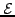and the variance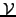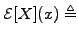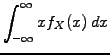(1)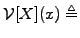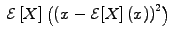(2)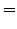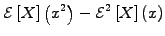(3)

where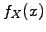is the density distribution function of a stochastic variable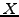. The notation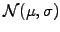refers to the set of Gaussian or normally distributed stochastic variablewith a density distribution function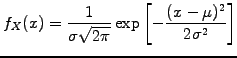(4)

where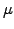is the mean and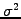is the variance. Along the same lines,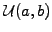stands for the set of uniformly distributed random numbers in the interval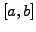.

Now consider a class of stochastic processes that play a central role in the Monte Carlo method: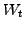is called a Wiener process (or in an equivalent manner Brownian motion) at a time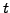if and only if

1.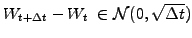, where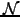is the set of normal distributed random numbers.
2.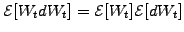, wheredenotes expected value,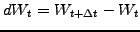and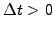, i.e. the Wiener increment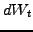is independent of the past.

It turns out that the distribution of Wiener increments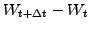is essentially the same function as the Green's function of the diffusion equation (1.3.2#eq.3).

The differential calculus of stochastic processes (or Itô calculus) involves new properties that are fundamentally different from the ordinary (or Riemann) calculus. The reason can be tracked down to the preferred direction of timein the Itô integral, which is defined as the limit of the explicit (forward Euler) discretization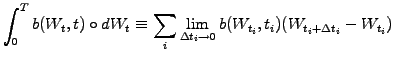(5)

for any sequence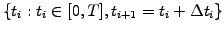. Note that the circle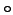here stands for Itô differential and states thatis independent of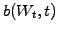; also, an implicit discretization in (5.2#eq.5) would give a fundamentally different result.

Define a property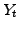that is a sum of a Riemann and an Itô integral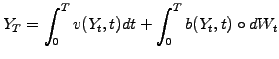(6)

For an infinitely short time interval, this leads to the stochastic differential equation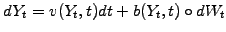(7)

which reduces to an ordinary differential equation in the absence of the stochastic component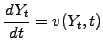(8)

Stochastic differential equations such as (5.2#eq.7) are used in the Monte-Carlo method to describe the trajectories or orbits of particles.

SYLLABUS  Previous: 5.1 Monte Carlo integration  Up: 5 MONTE-CARLO METHOD  Next: 5.3 Particle orbits